# Overview of Cost – Corporate and Management Accounting MCQ

Going through the Overview of Cost – Corporate and Management Accounting CS Executive MCQ Questions with Answers you can quickly revise the concepts.

## Overview of Cost – Corporate and Management Accounting MCQs

Question 1.
Which of these is not an objective of Cost Accounting?
(A) Ascertainment of cost
(B) Determination of selling price
(C) Cost control and cost reduction
(D) Assisting shareholders in decision making
(D) Assisting shareholders in decision making

Question 2.
A profit centre is a centre
(A) Where the manager has the responsibility of generating and maximising profits
(B) Which is concerned with earning an adequate Return on Investment
(C) Both of the above
(D) Which manages cost
(A) Where the manager has the responsibility of generating and maximising profits

Question 3.
Responsibility centre can be categorised into:
(A) Cost centres only
(B) Profit centres only
(C) Investment centres only
(D) Cost centres, profit centres and investment centres
(D) Cost centres, profit centres and investment centres

Question 4.
Cost Unit is defined as:
(A) Unit of quantity of product, service or time in relation to which costs may be ascertained or expressed
(B) A location, person or an item of equipment or a group of these for which costs are ascertained and used for cost control
(C) Centres having the responsibility of generating and maximising profits
(D) Centres concerned with earning an adequate return on investment
(A) Unit of quantity of product, service or time in relation to which costs may be ascertained or expressed

Question 5.
Fixed cost is a cost:
(A) Which changes in total in proportion to changes in output
(B) Which is partly fixed and partly variable in relation to output
(C) Which do not change in total during a given period despite changes in output
(D) Which remains same for each unit of output
(C) Which do not change in total during a given period despite changes in output

Question 6.
Uncontrollable costs are the costs which be influenced by the action of a specified member of an undertaking
(A) Cannot
(B) Can
(C) May or may not
(D) Must
(A) Cannot

Question 7.
Element/s of Cost of a product are:
(A) Material only
(B) Labour only
(C) Expenses only
(D) Material, Labour & Expenses
(D) Material, Labour & Expenses

Question 8.
Abnormal cost is the cost:
(A) Cost normally incurred at a given level of output
(B) Cost not normally incurred at a given level of output
(C) Cost which is charged to customer
(D) Cost which is included in the cost of the product
(B) Cost not normally incurred at a given level of output

Question 9.
Conversion cost includes cost of converting ………. into …………
(A) Raw material, WIP
(B) Raw material, Finished goods
(C) WIP, Finished goods
(D) Finished goods, Saleable goods
(B) Raw material, Finished goods

Question 10.
Sunk costs are ….
(A) Relevant for decision making
(B) Not relevant for decision making
(C) Cost to be incurred in future
(D) Future costs
(B) Not relevant for decision making

Question 11.
Describe the method of costing to be applied in case of Nursing Home:
(A) Operating Costing
(B) Process Costing
(C) Contract Costing
(D) Job Costing
(A) Operating Costing

Question 12.
Describe the cost unit applicable to the Bicycle industry:
(A) Per part of bicycle
(B) Per bicycle
(C) Per tonne
(D) Per day
(B) Per bicycle

Question 13.
Cost accounting
(A) Deals with historic data
(B) Has futuristic in approach
(C) Both (A) & (B)
(D) Neither (A) nor (B)
(B) Has futuristic in approach

Question 14.
Which of the following is feature of job costing?
(A) Single order
(B) Single contract
(C) Every job is cost unit itself
(D) All of the above
(D) All of the above

Question 15
……….. may be defined as the technique of presenting cost data where in variable costs and fixed costs are shown separately for managerial decision-making.
(A) Direct Costing
(B) Absorption Costing
(C) Marginal Costing
(D) Variance Analysis
(C) Marginal Costing

Question 16.
In which of the following cost accounting has do not have any role?
(A) Price fixation
(B) Inventory control
(C) Service sector
(D) Price earning
(D) Price earning

Question 17.
……….. is form of specific order costing which applies where work is undertaken as per customer’s specific requirement.
(A) Batch costing
(B) Operation costing
(C) Job costing
(D) Composite costing
(C) Job costing

Question 18.
Maintenance department in a factory is a ………..
(A) Profit Center
(B) Investment Centre
(C) Cost Driver
(D) Service Centre
(D) Service Centre

Question 19.
Process Costing is generally adopted by:
(A) Chemical industries
(B) Oil refineries
(C) Soap manufacturing
(D) All of the above
(D) All of the above

Question 20.
Costs of direct material, direct labour and direct expenses can be directly allocated or identified with a particular cost centres or a cost unit are the examples of …………
(A) Common Costs
(B) Indirect Cost
(C) Differential Costs
(D) Traceable Costs
(D) Traceable Costs

Question 21
………….. method of costing is used in large scale contract, as in case of building construction.
(A) Composite Costing
(B) Multiple Costing
(C) Operation Costing
(D) Contract Costing
(D) Contract Costing

Question 22.
Indirect costs are also known as …………..
(A) Common Costs
(B) Traceable Costs
(C) Implicit Costs
(D) Sunk Costs
(A) Common Costs

Question 23
……..is a specialized system of job costing applied to long-term contracts.
(A) Composite Costing
(B) Batch Costing
(C) Direct Costing
(D) Contract costing
(D) Contract costing

Question 24
…….. is the name given to the technique whereby standard costs are pre-determined and subsequently compared with the recorded actual costs.
(A) Direct Costing
(B) Absorption Costing
(C) Marginal Costing
(D) Variance Analysis
(D) Variance Analysis

Question 25
…………. represents the resources that have been sacrificed to attain a particular objective.
(A) Expense
(B) Cost
(C) Assets
(D) Liability
(B) Cost

Question 26.
Indirect costs are ………… to cost centres or cost units.
(A) Allocated
(B) Apportioned
(C) Aggregated
(D) Allowed
(B) Apportioned

Question 27.
Standard costing is not suitable ……..
(A) Where the manufacturing method involves production of standardised goods of repetitive nature
(B) Manufacturing organization producing different types of products
(C) Organizations engaged in service sector
(D) All of the above
(C) Organizations engaged in service sector

Question 28
method of costing is used when it is desired to ascertain the cost of carrying out an operation in a department.
(A) Operating Costing
(B) Operation Costing
(C) Composite Costing
(D) Process Costing
(B) Operation Costing

Question 29.
Which of the following is not objective of cost accounting?
(A) To arrive at the cost of production of every unit, job, operation, process.
(B) To provide information to enable management to make short-term decisions.
(C) To present true & fair view of overall results of the transactions, and events.
(D) None of the above
(C) To present true & fair view of overall results of the transactions, and events.

Question 30.
A company presently does not utilise its available capacity. In case of full capacity utilisation, the cost per unit shall
(A) Increase
(B) Decrease
(C) Remain constant
(D) None of the above
(B) Decrease

Question 31.
Service costing is also known as
(A) Operation Costing
(B) Operating Costing
(C) Direct Costing
(D) Uniform Costing
(B) Operating Costing

Question 32.
One of the most important technique in cost planning is :
(A) Direct Cost
(B) Budget
(C) Cost Sheet
(D) Marginal Costing
(D) Marginal Costing

Question 33.
CIMA, London defines as, “the establishment of budgets, standard costs and actual costs of operations, processes, activities or products; and the analysis of variances, profitability or the social use of funds”
(A) Management Accounting
(B) Standard Costing
(C) Budgetary Control
(D) Cost Accounting
(D) Cost Accounting

Question 34.
The variable cost per unit is:
(A) Variable in nature
(B) Fixed in nature
(C) Semi-variable in nature
(D) None of the above
(B) Fixed in nature

Question 35.
Which of the following difficulty is not faced at the time installation of costing system in any organization?
(A) Lack of support from top management
(B) Resistance from the existing staff
(C) Keen competition in oversea market
(D) All of the above
(C) Keen competition in oversea market

Question 36.
The total cost for producing 10 items is ₹ 15 and that for producing 15 items is ₹ 20. What is the fixed cost?
(A) ₹10
(B) ₹ 15
(C) ₹ 5
(D) None of the above
(C) ₹ 5

Question 37.
Cost accounting
(A) Present true & fair view of overall results of the transactions, and events.
(B) Uses monetary information only
(C) Both (A) & (B)
(D) Neither (A) nor (B)
(D) Neither (A) nor (B)

Question 38.
The main purpose of cost accounting is to:
(A) Maximise profits
(B) Help in inventory valuation
(C) Provide information to management for decision making
(D) Aid in the fixation of selling price
(C) Provide information to management for decision making

Question 39.
Direct costing technique can be applied:
(A) Stock valuation
(B) Determining whether to make or buy the product
(C) Close down decisions – like closing down of a department or shop
(D) All of the above
(D) All of the above

Question 40.
The CIMA, London, defines a …………………….. as, “a unit of product or service in relation to which costs are ascertained.”
(A) Cost Unit
(B) Cost Object
(C) Profit Center
(D) Cost Centres
(A) Cost Unit

Question 41.
The cost accountant is intimately connected with the …………..
(A) Production
(B) Marketing
(C) Finance
(D) All of the above
(D) All of the above

Question 42.
The term ……. is defined as the amount at any given volume of output by which aggregate costs are changed if the volume of output is increased or decreased by one unit.
(A) Differential Cost
(B) Marginal Cost
(C) Relevant Cost
(D) Incremental Cost
(B) Marginal Cost

Question 43.
Which of the following cannot be treated as are direct beneficiary of cost accounting?
(A) Management
(B) Debtors
(C) Employees
(D) Shareholders
(D) Shareholders

Question 44.
Pre-determined costs may be either:
(A) Standard cost
(B) Estimated cost
(C) Standard cost or estimated cost
(D) Estimated cost but not a standard cost
(C) Standard cost or estimated cost

Question 45.
Who may by order direct cost audit of a company, if in its opinion it is necessary to do so?
(A) The Ministry of Corporate Affairs
(B) The Parliament
(C) The Central Government
(D) All of the above
(C) The Central Government

Question 46.
Which of the following statement is false?
(A) The use of the term cost without qualification is not misleading.
(B) Fixed Costs are also called ‘traceable costs’
(C) Marginal cost is a predetermined calculation of how much costs should be under specified working conditions.
(D) All of the above
(D) All of the above

Question 47.
Which of the following is not method of costing?
(A) Uniform Costing
(B) Operating Costing
(C) Operation Costing
(D) Contract Costing
(A) Uniform Costing

Question 48.
If financial records can yield all the necessary costing information, it is not necessary to have a separate costing department.
(A) False
(B) True
(C) Partly correct
(D) Partly incorrect
(B) True

Question 49.
A ………. is a planned cost for a unit of product of service rendered.
(A) Standard cost
(B) Marginal Cost
(C) Opportunity Cost
(D) Historical Cost
(A) Standard cost

Question 50.
………… is defined as, “the techniques and process of ascertaining costs”.
(A) Cost Accounting
(B) Management Accounting
(C) Costing
(D) Cost Ascertainment
(C) Costing

Question 51.
Historical cost is the actual cost, determined
(B) After the event
(C) Estimated basis
(D) By applying common sense
(B) After the event

Question 52.
A power house in a factory is a ………….
(A) Service Centre
(B) Production Centre
(C) Profit Center
(D) Impersonal Cost Centre
(A) Service Centre

Question 53.
The management accounting data is derived from the ……….
(A) Cost accounting
(B) Financial accounting
(C) Cost & financial accounting
(D) Financial management
(C) Cost & financial accounting

Question 54
………. are those cost, which can be identified easily and indisputably with a unit of operation or costing unit or cost centre.
(A) Traceable Costs
(B) Common Costs
(C) Indirect Costs
(D) Historical Cost
(A) Traceable Costs

Question 55.
Service costing is used by
(A) Power generation
(B) Hospitals
(C) Transport companies
(D) All of the above
(D) All of the above

Question 56.
If costs are classified “By behaviour”, then which of the following is the correct classification?
(A) FixedCost,VariableCost & Semi-Variable Cost
(B) Historical Cost, Pre-Determined Cost, Standard Cost, Estimated Cost
(C) Production Cost, Administration Cost, Selling Cost, Distribution Cost, Research & Development Cost.
(A) FixedCost,VariableCost & Semi-Variable Cost

Question 57.
Which of the following report is not generated by cost accounting?
(A) Consumption of material statements
(B) Labour utilization statements
(C) Earnings per share
(D) Cost sheets
(C) Earnings per share

Question 58.
Costs of indirect material, indirect labour and indirect expenses in aggregate constitute the ………..
(B) Historical Costs
(C) Pre-determined Costs
(D) Estimated Costs

Question 59
…….. is a pre-determined cost based on past performance adjusted to anticipated changes.
(A) Te-be Veimme
(B) Estimated Costs
(C) Differential Cost
(D) Historical Costs
(B) Estimated Costs

Question 60.
Which of the following is purpose of cost accounting?
(A) It is more attached with reporting the results and position of business.
(B) It aims at classifying and summarizing in significant manner and in terms of money, transaction and events, which are, in part at least, of a monetary in nature and interpreting the result thereof.
(C) Both (A) & (B)
(D) Neither (A) nor (B)
(D) Neither (A) nor (B)

Question 61.
Increase in total variable cost is due to:
(A) Increase in fixed cost
(B) Increase in sales
(C) Increase in production
(D) All of the above
(C) Increase in production

Question 62.
Indirect costs to cost units or cost centres and have to be absorbed or recovered into cost units are termed as indirect costs.
(A) Cannot be directly allocated
(B) Cannot be directly apportioned
(C) Both (A) & (B)
(D) Either (A) or (B)
(A) Cannot be directly allocated

Question 63.
CIMA, London defines as, “the establishment of budgets, standard costs and actual costs of operations, processes, activities or products; and the analysis of variances, probability or the social use of funds”
(A) Management Accounting
(B) Financial Accounting
(C) Cost Accounting
(D) Financial Management
(C) Cost Accounting

Question 64.
…………. is the aggregate cost related to a cost unit, which consists of a group of similar articles which maintain its identity throughout one or more stages of production.
(A) Pre-determined Costs
(B) Batch Cost
(C) Process Cost
(D) Operation Cost
(B) Batch Cost

Question 65.
Which of the following statement is false?
(A) In most of the cases cost accounting system is installed on grounds of its benefits.
(B) Costing helps in making estimates
(C) Costing helps in determining and enhancing efficiency.
(D) The existing financial accounting staff may not resist to the costing system.
(D) The existing financial accounting staff may not resist to the costing system.

Question 66.
Interest paid on own capital not involving any cash outflow is example of:
(A) Product Cost
(B) Opportunity Cost
(C) Imputed Cost
(D) Explicit Cost
(C) Imputed Cost

Question 67.
An example of fixed cost is:
(A) Direct material cost
(B) Works manager’s salary
(C) Depreciation of machinery
(D) Chargeable expenses
(B) Works manager’s salary

Question 68.
…….. is the name given to a common system of costing followed by number of firms in the same industries.
(A) Common Costing
(B) Absorption Costing
(C) Direct Costing
(D) Uniform Costing
(D) Uniform Costing

Question 69.
Differential costs are also known as ………………
(A) Incremental Cost
(B) Opportunity Cost
(C) Period Cost
(D) Controllable Cost
(A) Incremental Cost

Question 70.
The cost audit order can be given by the Central Government only in respect of Class of Companies which is required to maintain books of account under the provisions of …….. of the Companies Act, 2013.
(A) Section 148
(B) Section 138
(C) Section 209(1)(d)
(D) Section 128
(A) Section 148

Question 71.
“Rent paid for the factory building which is temporarily closed” is example of
(A) Imputed cost
(B) Sunk cost
(C) Shut down cost
(D) Temporary cost
(C) Shut down cost

Question 72.
Cost accounting system can be installed without management accounting.
(A) False
(B) True
(C) Partly incorrect
(D) None of the above
(B) True

Question 73.
……………. is the cost of selecting one course of action and the loosing of the opportunities to carry out other course of action.
(A) Replacement Cost
(B) Hypothetical Cost
(C) Explicit Cost
(D) Opportunity Cost
(D) Opportunity Cost

Question 74.
If costs are classified “by time’’, then which of the following is the correct classification?
(A) Marginal Cost, Differential Cost, Opportunity Cost, Replacement Cost, Relevant Cost, Imputed Cost, Sunk Cost, Normal Cost, Abnormal Cost, Avoidable Cost, Unavoidable Cost etc.
(B) Historical Cost, Pre-Determined Cost, Standard Cost, Estimated Cost
(C) Production Cost, Administration Cost, Selling Cost, Distribution Cost, Research & Development Cost.
(B) Historical Cost, Pre-Determined Cost, Standard Cost, Estimated Cost

Question 75.
Cost of goods produced includes:
(A) Production cost and finished goods inventory
(B) Production cost and work-in-progress
(C) Production cost, work-in-progress and finished goods inventory
(D) None of the above
(B) Production cost and work-in-progress

Question 76.
Which of the following “cost unit” is suitable for Bicycle industries?
(A) Number
(B) Per kilogram
(C) Per tonne
(D) Per square foot
(A) Number

Question 77.
Conversion Cost = …………….
(A) Direct wages + Direct expenses
(B) Direct wages + Direct expenses + Manufacturing overhead
(C) Direct material + Direct wages + Direct expenses + Manufacturing overhead
(B) Direct wages + Direct expenses + Manufacturing overhead

Question 78.
When a new factory is stared or when a new product is introduced, certain expenses are incurred. There are trial runs. Such costs are termed as ………… and treated as deferred revenue expenditure.
(A) Pre-production costs
(B) Preliminary expenses
(C) Prepaid costs
(D) Capital loss
(A) Pre-production costs

Question 79.
Conversion cost is equal to the total of:
(A) Material cost and direct wages
(B) Material cost and indirect wages
(C) Direct wages and factory overhead
(D) Material cost and factory overhead
(C) Direct wages and factory overhead

Question 80
………….. are also known as out of pocket costs.
(A) Explicit Costs
(B) Implicit Costs
(C) Notional Costs
(D) Economic Costs
(A) Explicit Costs

Question 81.
………….. are the costs, which have been created by a decision that was made in the past and cannot be changed by any decision that will be made in the future.
(A) Sunk Costs
(B) Historical Costs
(C) Past Costs
(D) All of the above
(D) All of the above

Question 82.
Which of the following cost is controllable cost?
(A) Fixed Costs
(B) Variable Costs
(C) Fixed Costs & Indirect Costs
(D) Period Costs
(B) Variable Costs

Question 83.
Which of the following “cost unit” are suitable for chemical industries?
(A) Per meter, Per square foot, Ream
(B) Per gross, Per gallon, Per dozen bottle
(C) Liter, gallon, kilograms, tonne
(D) None of the above
(C) Liter, gallon, kilograms, tonne

Question 84.
………….. is normally incurred at a given level of output in the conditions in which that level of output is achieved.
(A) Normal cost
(B) Abnormal Cost
(C) Process Cost
(D) All of the above
(A) Normal cost

Question 85.
A company intends to rearrange production facilities, of future costs are as under:

 Item of cost Existing Proposed rearrange­ment Direct material 10.00 10.00 per unit Direct labour 5.00 4.00 per unit

With reference to above data which cost is relevant cost for a company?
(A) Direct material cost
(B) Direct labour cost
(C) Both (A) & (B)
(D) Either (A) or (B)
(B) Direct labour cost

Question 86.
According to CIMA, A cost which can be influenced by its budget holder is known as
(A) Controllable Costs
(B) Notional Costs
(C) Uncontrollable Costs
(D) Fixed Costs
(A) Controllable Costs

Question 87.
…… is any sub-unit of an organization to which both revenues and costs are assigned, so that the responsibility of a sub-unit may be measured.
(A) Profit Centre
(B) Cost Centre
(C) Process Cost Centre
(D) Investment Centre
(A) Profit Centre

Question 88.
……………. are not recorded in the books of account.
(A) Implicit Costs
(B) Explicit Costs
(C) Sunk Costs
(D) All of the above
(A) Implicit Costs

Question 89.
Which of the following “cost unit” is not used by the organization engaged in providing services?
(A) Tonne km
(B) Passenger km
(C) Kilowatt hour
(D) Per meter
(D) Per meter

Question 90.
Product costs are ……………
(A) Fixed in nature.
(B) Related period, hence also known as indirect cost.
(C) Not controllable.
(D) Also known as direct cost.
(D) Also known as direct cost.

Question 91.
………. is an unusual or a typical cost
whose occurrence is usually irregular and unexpected.
(A) Normal Cost
(B) Abnormal Cost
(C) Budgeted Cost
(D) All of the above
(B) Abnormal Cost

Question 92.
In which of the following industries “contract costing” is used?
(A) Ship building
(B) Nursing home
(C) Textile mills
(D) Bicycle
(A) Ship building

Question 93.
………. is a variable cost of one unit of a product or a service ie., a cost which would be avoided if that unit was not produced or provided.
(A) Differential Cost
(B) Incremental Cost
(C) Replacement Cost
(D) Relevant Cost
(D) Relevant Cost

Question 94.
………… are the costs incurred in relation to the temporary closing or a department/ division/enterprise.
(A) Start up costs
(B) Shutdown costs
(C) Temporary costs
(D) Relevant costs
(B) Shutdown costs

Question 95.
Costs which are ascertained after they have been incurred are known as …………
(A) Imputed costs
(B) Sunk costs
(C) Historical costs
(D) Opportunity costs
(C) Historical costs

Question 96.
Prime costs plus variable overhead is known as:………….
(A) Production cost
(B) Marginal costs
(C) Total cost
(D) Cost of sales
(A) Production cost

Question 97.
Notional costs are considered in …………..
(A) Financial Accounting
(B) Cost Accounting
(C) Both (A) & (B)
(D) Neither (A) nor (B)
(B) Cost Accounting

Question 98.
Costs that change in response to alternative courses of action are called:
(A) Relevant costs
(B) Differential costs
(C) Target costs
(D) Sunk costs
(B) Differential costs

Question 99.
Which of the following method of costing is suitable for “Interior decoration”?
(A) Job Costing
(B) Operating Costing
(C) Multiple Costing
(D) Batch Costing
(A) Job Costing

Question 100.
…………. are also known as accounting costs.
(A) Explicit Costs
(B) Implicit Costs
(C) Notional Costs
(D) Opportunity costs
(A) Explicit Costs

Question 101.
Indirect costs are known as:
(A) Variable costs
(B) Fixed costs
(D) None of the above

Question 102.
If ₹ 10 is spend on producing 10 units and ₹ 15 for producing 15, then the fixed cost per unit is:
(A) ₹ 0
(B) ₹ 1
(C) ₹ 2
(D) ₹ 5
(A) ₹ 0

Question 103.
The main difference between the profit centre and investment centre is:
(A) Decision making
(B) Revenue generation
(C) Cost incurrence
(D) Investment
(A) Decision making

Question 104.
A division of company, which produces and markets the product, can be called as …….
(A) Profit Centre
(B) Process Centre
(C) Service Centre
(D) Investment Centre
(A) Profit Centre

Question 105.
Which of the following is not a relevant cost?
(A) Replacement cost
(B) Sunk cost
(C) Marginal cost
(D) Standard cost
(B) Sunk cost

Question 106.
Which of the following cost is linked with the calculation of cost of inventories?
(A) Product Cost
(B) Period Cost
(C) Both Product & Period Cost
(D) Historical Cost
(A) Product Cost

Question 107.
All of the following are features of a relevant cost except:
(A) They affect the future cost
(B) They cause an increment in cost
(C) Relevant cost is a sunk cost
(D) They affect the future cash flows
(C) Relevant cost is a sunk cost

Question 108.
Which of the following method of costing is suitable for “Airline Company”?
(A) Job Costing
(B) Operating Costing
(C) Multiple Costing
(D) Batch Costing
(B) Operating Costing

Question 109.
Which of the following statement is TRUE about the relevant cost?
(A) It is a sunk cost
(B) It is an opportunity cost
(C) It do not affect the decision making process
(D) All costs are relevant
(B) It is an opportunity cost

Question 110.
The cost of electricity bill of the factory is treated as:
(A) Fixed cost
(B) Variable cost
(C) Step cost
(D) Semi variable cost
(D) Semi variable cost

Question 111.
A firm uses its own capital or uses its owner’s time and/or financial resources both are examples of ……….
(A) Implicit Cost
(B) Explicit Cost
(C) Sunk Cost
(D) Relevant Cost
(A) Implicit Cost

Question 112.
Which of the following would be considered to be an investment centre?
(A) Managers have control over marketing
(B) Managers have a sales team
(C) Managers have a sales team and are given a credit control function
(D) Managers can purchase capital assets and are given a credit control function
(D) Managers can purchase capital assets and are given a credit control function

Question 113.
The total cost incurred in the operation of a business undertaking other than the cost of manufacturing and production is known as………..
(A) Direct cost
(B) Variable cost
(C) Commercial cost
(D) Conversion cost
(C) Commercial cost

Question 114.
If costs are classified by nature, then which of the following is the correct classification?
(A) Marginal Cost, Differential Cost, Opportunity Cost, Replacement Cost, Relevant Cost, Imputed Cost, Sunk Cost, Normal Cost, Abnormal Cost, Avoidable Cost, Unavoidable Cost etc.
(B) Historical Cost, Pre-Determined Cost, Standard Cost, Estimated Cost
(C) Production Cost, Administration Cost, Selling Cost, Distribution Cost, Research & Development Cost.
(D) Material, Labour, Expenses
(D) Material, Labour, Expenses

Question 115.
In decision making all costs already incurred in past should always be:
(A) Ignored
(B) Considered
(C) Partially ignored
(D) Partially considered
(A) Ignored

Question 116.
Cost accounting concepts include all of the following except:
(A) Planning
(B) Controlling
(C) Sharing
(D) Costing
(C) Sharing

Question 117.
Which of the following statement is TRUE about historical cost?
(A) It is always relevant to decision making
(B) It is always irrelevant to decision making
(C) It is always an opportunity cost
(D) It is always realizable value
(B) It is always irrelevant to decision making

Question 118.
Colin Drury defined as, “any activity for which a separate measurement of costs is desired”.
(A) Cost Driver
(B) Cost Centre
(C) Cost Object
(D) Profit Center
(C) Cost Object

Question 119.
Expenses such as rent and depreciation of a building are shared by several departments these are:
(A) Indirect expenses
(B) Direct expenses
(C) Joint expenses
(D) All of the above
(A) Indirect expenses

Question 120.
Period costs are:
(A) Expensed when the product is sold
(B) Included in the cost of goods sold
(C) Related to specific period
(D) Not expensed
(C) Related to specific period

Question 121.
All of the following compose cost of goods sold except:
(A) Raw material
(B) Labour
(C) Capital
(C) Capital

Question 122.
…………. can be influenced by the action of the executive heading the responsibility center.
(A) Fixed Costs
(B) Uncontrollable Costs
(C) Controllable Costs
(D) Unavoidable Costs
(C) Controllable Costs

Question 123.
CIMA defines as, a production or service, function, activity or item of equipment whose costs may be attributed to cost units.
(A) Cost Driver
(B) Cost Centre
(C) Investment Centre
(D) Profit Center
(B) Cost Centre

Question 124.
A segment of the business that generates both revenue and cost is called:
(A) Profit centre
(B) Cost centre
(C) Cost driver
(D) All of these
(A) Profit centre

Question 125.
What will be the impact of normal loss on the overall per-unit cost?
(A) Per unit cost will increase
(B) Per unit cost will decrease
(C) Per unit cost remain unchanged
(D) Normal loss has no relation to unit cost|
(A) Per unit cost will increase

Question 126.
A fixed cost:
(A) May change in total when such change is not related to changes in production
(B) Will not change in total because it is not related to changes in production
(C) Is constant per unit for each unit of change in production
(D) May change in total, depending on production with the relevant range
(B) Will not change in total because it is not related to changes in production

Question 127.
All of the following are the features of fixed costs except:
(A) Although fixed within a relevant range of activity level but are relevant to a decision making when it is avoidable.
(B) Although fixed within a relevant range of activity level but are relevant to a decision making when it is incremental.
(C) Generally it is irrelevant
(D) It is relevant to decision making under any circumstances
(D) It is relevant to decision making under any circumstances

Question 128
…………. are constant per unit but change in totality.
(A) Fixed Costs
(B) Semi-variable Costs
(C) Uncontrollable Costs
(D) Variable Costs
(D) Variable Costs

Question 129.
The basic assumption made with respect to fixed costs is that
(A) Fixed cost is a controllable cost
(B) Fixed cost is a product cost
(C) Fixed cost is an irrelevant cost
(D) Fixed cost is a period cost
(D) Fixed cost is a period cost

Question 130.
Which of the following is NOT a relevant cost to decision making?
(A) Opportunity costs
(B) Relevant benefits
(C) Avoidable costs
(D) Sunk costs
(D) Sunk costs

Question 131.
Which of the following product cost is Included in prime cost and conversion cost?
(A) Direct labour
(C) Direct material
(D) Work in Process
(A) Direct labour

Question 132.
Which of the following costs would NOT be a period cost?
(A) Indirect materials
(D) Selling costs
(A) Indirect materials

Question 133.
Management Accounting seeks to serve the purpose of management to run a business more efficiently and thus uses the techniques of:
(A) Financial Accounting
(B) Cost Accounting
(C) Mathematics and Statistics
(D) All of the above
(D) All of the above

Question 134.
Which of the following is not a method of costing?
(A) Marginal costing
(B) Job costing
(C) Process costing
(D) Operating costing
(A) Marginal costing

Question 135.
Which of the following is not a Technique of costing?
(A) Absorption costing
(B) Standard costing
(C) Multiple costing
(D) Marginal costing
(C) Multiple costing

Question 136.
A cost centre is:
(A) A unit of production in relation to which costs are ascertained
(B) A location which is responsible for controlling direct costs
(C) Any location or department which incurs cost
(D) None of these
(A) A unit of production in relation to which costs are ascertained

Question 137.
A cost centre is:
(A) A unit of product or service in relation to which costs are ascertained
(B) An amount of expenditure attributable to an activity
(C) A production or service location, function, activity or item of equipment for which costs are accumulated
(D) A centre for which an individual budget is drawn up
(A) A unit of product or service in relation to which costs are ascertained

Question 138.
Cost centre may be –
(A) Location
(B) Person
(C) Item of equipment
(D) Any of the above
(D) Any of the above

Question 139.
A ……….. is a planned cost for a unit of product of service rendered.
(A) Standard cost
(B) Marginal Cost
(C) Opportunity Cost
(D) Historical Cost
(A) Standard cost

Question 140.
Raw materials directly identifiable as part of the final product is classified as:
(A) Period costs
(B) Fixed costs
(C) Direct materials
(D) Any of the above
(C) Direct materials

Question 141.
Inventory consists of ……….
(A) Intangible property
(B) Tangible property
(C) (A)or(B)
(D) (A) & (B)
(B) Tangible property

Question 142.
Which of the following statement is correct in relation to “Need for proper inventory control”?
(B) Materials do not constitute a significant part of the total production cost hence proper planning and controlling of inventories is not a big deal.
(C) Funds are not tied up in surplus stores and stocks.
(D) All of the above

Question 143.
Inventory is valued at ……..
(A) Replacement price
(B) Replacement price or purchase value, whichever is less.
(C) At cost or net realizable value which-ever is less.
(D) Replacement price or net realizable value, whichever is less.
(C) At cost or net realizable value which-ever is less.

Question 144.
Inventory held for sale in the ordinary course of business is known as ………….
(A) Finished Goods
(B) Raw Material
(C) Work-in-progress
(D) Miscellaneous inventory
(A) Finished Goods

Question 145.
Which of the following method is based on the assumption that, latest consignment of a materials or goods manufactured are exhausted first and the closing stock is valued at the cost of earliest lot in hand?
(A) FIFO Method
(B) Highest-in-first-out method
(C) Average cost method
(D) LIFO Method
(D) LIFO Method

Question 146.
………….. are those cost, which can be identified and traceable to particular product or costing unit or cost centre.
(A) Indirect material costs
(B) Period costs
(C) Direct material costs
(D) Fixed costs
(C) Direct material costs

Question 147.
Wood used in production of tables and chairs, steel bars used in steel factory etc. are the examples of ………..
(A) Indirect material
(B) Direct material
(C) Fixed material
(D) All of the above
(B) Direct material

Question 148.
……….. are those items, which are moving at a slow rate and this may arise due to general depression in demand due to keen competition.
(A) Dormant stocks
(B) Written-off stocks
(C) Slow moving stocks
(D) Any of the above
(C) Slow moving stocks

Question 149.
………… are those items which are not moving temporarily but their movement is expected shortly.
(A) Slow moving stock
(B) Dormant stocks
(C) Non-marketable stock
(D) Less efficient stock
(B) Dormant stocks

Question 150.
If small quantities of direct material used in the end product like gums and threads are used in binding books then it may be categorized as
(A) Miscellaneous cost
(B) Preliminary cost
(C) Indirect material cost
(D) Fixed cost of production
(C) Indirect material cost

Question 151.
Which of the following technique can be used for inventory control?
(A) Standard Costing
(B) ABC Analysis
(C) Integrated Accounting System
(D) Any of the above
(B) ABC Analysis

Question 152.
……….. is an optimum quantity of material to be ordered every time an order is placed. EOQ may be defined as that quantity of purchase which minimizes material order cost and material carrying cost.
(A) Quantity in such lot which has maximum discount
(B) Special Order Quantity (SOQ)
(C) Standard Order Quantity (SOQ)
(D) Economic Order Quantity (EOQ)
(D) Economic Order Quantity (EOQ)

Question 153.
The model and formula of EOQ was developed by in 1913.
(A) F.W. Taylor
(B) F. Wilson Harris
(C) F. Walter Harris
(D) F.W. Marshall
(B) F. Wilson Harris

Question 154.
Under ………… a continuous record of receipt and issue of materials is maintained by the stores department and the information about the stock of material is always available.
(A) Perpetual Inventory System
(B) Continuous Stock Taking
(C) Periodic Inventory System
(D) Just in time
(A) Perpetual Inventory System

Question 155.
purchase means the purchase of goods or material such that delivery immediately precedes their use.
(A) Economic order quantity
(B) Reorder point
(C) Re-order Quantity
(D) Just in time (JIT)
(D) Just in time (JIT)

Question 156.
Out of the following, what is not the work of purchase department?
(A) Receiving purchase requisition
(B) Exploring the sources of material supply
(C) Preparation and execution of purchase orders

Question 157.
Which one out of the following is not an inventory valuation method?
(A) FIFO
(B) LIFO
(C) Weighted Average
(D) EOQ
(D) EOQ

Question 158.
In case of rising prices (inflation), FIFO method will:
(A) Provide lowest value of closing stock and profit
(B) Provide highest value of closing stock and profit
(C) Provide highest value of closing stock but lowest value of profit
(D) Provide highest value of profit but lowest value of closing stock
(B) Provide highest value of closing stock and profit

Question 159.
In case of rising prices (inflation), LIFO will:
(A) Provide lowest value of closing stock and profit
(B) Provide highest value of closing stock and profit
(C) Provide highest value of closing stock but lowest value of profit
(D) Provide highest value of profit but lowest value of closing stock
(A) Provide lowest value of closing stock and profit

Question 160.
The labour cost which is traceable or identified to particular product or cost centre is known as –
(A) Indirect labour cost
(B) Direct labour cost
(C) Variable labour cost
(D) (B) or (C)
(D) (B) or (C)

Question 161.
Rent free accommodation or accommodation provided at concessional rate should be classified as:
(A) Fringe Benefits
(B) Deferred Monetary Benefits
(C) Fixed standard labour cost
(D) Pecuniary Benefits
(A) Fringe Benefits

Question 162.
………. is process whereby company make assessment of particular job to know how worth is it.
(A) Merit Rating
(B) Performance appraisal
(C) Induction
(D) Job evaluation
(D) Job evaluation

Question 163.
Direct labour cost ……….
(A) Should be treated as production overhead.
(B) Should be charged using supplementary overhead rate
(C) Should be treated as abnormal cost and charged to costing profit & loss account.
(D) Becomes part of prime cost.
(D) Becomes part of prime cost.

Question 164.
Direct labour cost is in nature.
(A) Variable
(B) Fixed
(C) Semi-variable
(D) Fixed or semi-variable
(A) Variable

Question 165.
Which of the following is not objective of time-keeping?
(A) Preparation of payrolls
(B) Ascertaining idle time
(C) Ascertaining and controlling labour cost
(D) Disability, making a worker unfit for work
(D) Disability, making a worker unfit for work

Question 166.
In relation to labour cost “Production Bonus” will be classified as
(A) Pecuniary Benefits
(B) Deferred Monetary Benefits
(C) Fringe Benefits
(D) Non-Pecuniary Gains
(A) Pecuniary Benefits

Question 167.
When some benefit or allowances are given to employee in addition to normal wages/salary, then such additional benefits are popularly called as
(B) Deferred benefits
(C) Non-absorbed labour cost
(D) Fringe benefits
(D) Fringe benefits

Question 168.
…………. refers to correct recording of the employees’ attendance time.
(A) Time booking
(B) Timekeeping
(C) Time spending
(D) Time pass
(B) Timekeeping

Question 169.
(A) Direct or prime cost
(B) All indirect costs
(C) Only factory indirect costs
(D) Only indirect expenses
(B) All indirect costs

Question 170.
Allotment of whole item of cost to a cost centre or cost unit is known as
(A) Cost Apportionment
(B) Cost Allocation
(C) Cost Absorption
(D) Machine hour rate
(B) Cost Allocation

Question 171.
(A) Indirect Materials
(B) Indirect Labour
(C) Indirect Expenses
(D) All of the above
(D) All of the above

Question 172.
If an item of overhead expenditure is charged specifically to a single department this would be an example of:
(A) Apportionment
(B) Allocation
(C) Re-apportionment
(D) Absorption
(B) Allocation

Question 173.
The arrangement of various items of overhead costs in logical groups having regard to their nature is known as

Question 174
……….. implies the allotment of whole items of cost to cost centres or cost units whether it may be production cost centres or service cost centres.
(A) Apportionment
(B) Allocation
(C) Absorption
(D) Classification
(B) Allocation

Question 175.
The process of distribution of over-heads allotted to a particular department or cost centre over the units produced is called
(A) Allocation
(B) Apportionment
(C) Absorption
(D) Departmentalization
(B) Apportionment

Question 176.
If overheads are classified by “element wise” then which of the following classification is correct?
(C) Indirect materials, Indirect labour, Indirect expenses
(D) None of the above
(C) Indirect materials, Indirect labour, Indirect expenses

Question 177.
Cost of primary packing necessary for protecting the product or for convenient handling, should
(A) Become a part of the prime cost
(B) Become a part of the factory over-heads
(C) Charged to costing profit and loss account using supplementary over-head rate.
(D) Treated as selling and distribution overheads
(A) Become a part of the prime cost

Question 178.
If actual overheads are less than pre-determined overheads then it is case of:
(A) Under Absorption
(B) Over Absorption
(C) Low Absorption
(D) None of the above
(B) Over Absorption

Question 179.
If actual overheads are more than predetermined overheads then it is case of:
(A) Over Absorption
(B) Under Absorption
(C) (A) or (B)
(D) (A) & (B)
(B) Under Absorption

Question 180.
Opening Stock = 25,000 units, Closing Stock = 15,000 units. Purchases = 1,90,000 units. ( Take 1 year = 360 days). Stock Velocity = ?
(A) 30 days
(B) 90 days
(C) 60 days
(D) 36 days
(D) 36 days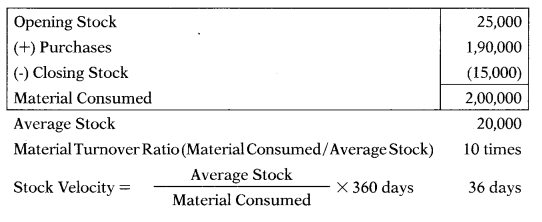Question 181.
Inventory turnover ratio = 2.5 times, Opening Stock=90,000 units, Closing Stock = 1,10,000 units. Purchases = ?
(A) 2,30,000 units
(B) 2,70,000 units
(C) 2,50,000 units
(D) 2,40,000 units
(B) 2,70,000 units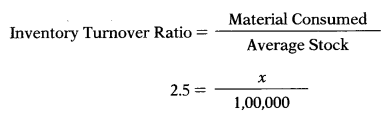Material Consumed = 2,50,000
Opening Stock + Purchases – Closing Stock = Material Consumed
90,000 + Purchases – 1,10,000 = 2,50,000
Purchases = 2,70,000

Question 182.
Stock Velocity =180 days, Material consumed = 1,50,000, Closing Stock = 62,500 units. Opening stock = ?
(A) 2,12,500 units
(B) 3,00,000 units
(C) 87,500 units
(D) 1,62,500 units
(C) 87,500 units

Question 183.
The following data relate to two activity levels of production :

 No. of units 4,500 5,750 Overheads (₹) 2,69,750 2,89,125

(A) ₹ 15.50
(B) ₹ 44.44
(C) ₹ 59.94
(D) None of the above
(A) ₹ 15.50

Question 184.
If you know that with 8 emits of output, average fixed cost is ₹ 12.50 and average variable cost is ₹ 81.25, then total cost at this output level is:
(A) ₹ 93.75
(B) ₹ 97.78
(C) ₹750
(D) ₹ 880
(C) ₹750

Question 185.
Following data has been extracted from the records of manufacturing company:
Total overhead for an activity level of 5,00,000 machine hours = ?

 Machine Hours 8,00,000 3,00,000 Overheads ₹52,00,000 ₹32,00,000

(A) ₹ 32,00,000
(B) ₹ 52,00,000
(C) ₹ 40,00,000
(D) ₹ 45,00,000
(C) ₹ 40,00,000

Question 186.
A management consultancy recovers overheads on chargeable consulting hours. Budgeted overheads were ₹ 6,15,000 and actual consulting hours were 32,150. Over-heads, were under-recovered by ₹ 35,000. If actual overheads, were ₹ 6,94,075, what was the budgeted overhead absorption rate per hour ?
(A) ₹ 19.13
(B) ₹ 20.50
(C) ₹ 21.59
(D) ₹ 22.68
(B) ₹ 20.50

Question 187.
Following data are made available by the company for the year ended 31.3.2019:
Factory cost = ₹ 10,57,736
Work-in-progress = ₹ 25,487
Machine hour = 4,188 hours
Absorption rate for absorption of production overhead = ?
(A) ₹ 121.02 per hour
(B) ₹ 252.36 per hour
(C) ₹ 373.58 per hour
(D) ₹ 83.21 per hour
(D) ₹ 83.21 per hour

Question 188.
The monthly budget of a department is as under:
Direct material : ₹ 45,000
Direct wages : ₹ 60,000
Direct labour hours : Hours 15,000
Machine hours : ₹ Hours 30,000
Find out the overhead recovery rate based on machine hours.
(A) 6 per hour
(B) 3 per hour
(C) 300%
(D) 50%
(B) 3 per hour

Question 189.
Following data relate to two output levels of a department:
Machine hours — 17,000 — 18,500
Overheads (₹) — 2,46,500 — 2,51,750
The variable overhead rate per hour = ?
(A) ₹ 5 per hour
(B) ₹ 4.5 per hour
(C) ₹ 4 per hour
(D) ₹ 3.5 per hour
(D) ₹ 3.5 per hour

Question 190.
Direct material=₹ 2,100, Direct wages = ₹ 660. Overhead absorption rate for Ma-chining, Assembly, Packing departments was respectively ₹ 4.5, ₹ 1.4, ₹ 2.5 per hour. Requires working of 180, 120 & 40 hours work in Machining, Assembly and Packing department respectively. The factory adds 30% on the factory cost to cover administration & selling overheads and profit. Selling price = ? .
(A) ₹ 3,585
(B) ₹ 4,661
(C) ₹ 4,989
(D) ₹ 5,262
(C) ₹ 4,989

Question 191.
B Ltd. estimated that during the year 75,000 machine hours would be used and it has been using an overhead absorption rate of ₹ 6.40 per machine hour in its machining department. During the year the overhead expenditure amounted to ₹ 4,72,560 and 72,600 machine hours were used. Which one of the following statements is correct?
(A) Overhead was under-absorbed by ₹ 7,440
(B) Overhead was under-absorbed by ₹ 7,920
(C) Overhead was over-absorbed by ₹ 7,440
(D) Overhead was over-absorbed by ₹ 7,920
(B) Overhead was under-absorbed by ₹ 7,920

Question 192.
A business always absorbs its overheads on labour hours. In the 8th period, 18,000 hours were worked, actual overheads were ₹ 2,79,000 and there was ₹ 36,000 over-absorption. The overhead absorption rate per hours was:
(A) ₹ 15.50
(B) ₹ 17.50
(C) ₹ 18.00
(D) ₹ 13.50
(B) ₹ 17.50

Question 193.
A company calculates the prices of jobs by adding overheads to the prime cost and adding 30% to total costs as a profit margin. Job number Y256 was sold for ₹ 1,690 and incurred overheads of ₹ 694. What was the prime cost of the job?
(A) ₹ 489
(B) ₹ 606
(C) ₹ 996
(D) ₹ 1,300
(B) ₹ 606

Question 194.
H Ltd. uses pre-determined overhead rates to apply manufacturing overhead to jobs. The pre-determined overhead rate is based on machine hours in the machining department and direct labour cost in the assembly department. At the beginning of the year, the company made the following estimates: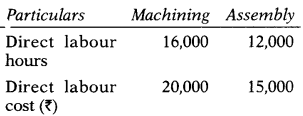What predetermined overhead rates would be used in the Machining and Assembly Departments, respectively.
(A) 110% and? 15
(B) ₹ 5.00 and 50%
(C) ₹ 8.00 and 50%
(D) ₹ 5.00 and 200%
(D) ₹ 5.00 and 200%

Question 195.
Job 21 was unfinished at the end of the accounting period. The total cost assigned to the job is ? 12,000 of which ₹ 3,000 is direct material. Factory overhead is allocated to goods in process at 150% of direct labour cost. What was the amount of direct labour charged to Job 21?
(A) ₹ 9,000
(B) ₹ 3,600
(C) ₹ 4,000
(D) ₹ 3,000
(B) ₹ 3,600

Question 196.
The production cost to produce one unit of finished goods was ₹ 45. Direct materials were 1/3 of the total cost, and direct labour was 40% of the combined total of direct labour and direct materials. The cost for direct materials, direct labour, and factory overhead was:
(A) ₹ 15, ₹ 18, & ₹ 12 respectively
(B) ₹ 15, ₹ 12, & ₹ 18 respectively
(C) ₹ 15, ₹ 16, & ₹ 14 respectively
(D) ₹ 15, ₹ 10, & ₹ 20 respectively
(D) ₹ 15, ₹ 10, & ₹ 20 respectively15 + 0.4% = %
15 = 0.6%
% = Prime Cost = 25

Question 197.
Following details are given to you:
Raw materials consumed ₹ 80,000
(A) ₹ 88,000
(B) ₹ 50,000
(C) ₹ 68,000
(D) ₹ 60,000
(B) ₹ 50,000

Question 198.
Dec 2014: The term used for ‘process of ascertaining the cost’ is known as ………..
(A) Cost
(B) Costing
(C) Cost accounting
(D) Cost accountancy
(B) Costing

Question 199.
Dec 2014: Which element of total cost is common in prime cost and conversion cost ………..
(C) Direct materials
(D) Direct labour
(D) Direct labour

Question 200.
Dec 2014: The cost that increases as the volume of activity decreases within the relevant range, is known as ………..
(A) Average cost per unit
(B) Average variable cost per unit
(C) Total fixed cost
(D) Total variable cost
(A) Average cost per unit

Question 201.
Dec 2014: Which of the following is generally used as cost unit of brick works ……..
(A) 1,000 bricks
(B) 100 bricks
(C) 10 bricks
(D) 1 brick
(A) 1,000 bricks

Question 202.
Dec 2014: Relevant costs are ……..
(A) Future costs
(B) Standard costs
(C) Controllable costs
(D) Historical costs
(A) Future costs

Question 203.
Dec 2014: Which of the following is not considered as a function of management accounting?
(A) Financial planning
(B) Decision making
(C) Reporting
(D) Cost computation
(B) Decision making

Question 204.
Dec 2014: The cost of selecting one course of action and forgoing the other is known as ………
(A) Sunk cost
(B) Differential cost
(C) Opportunity cost
(D) Joint cost
(C) Opportunity cost

Question 205.
Dec 2014: Expenditure on labour and materials that cannot be economically identified with a specific saleable cost unit is known as …….
(A) Prime cost
(C) Direct cost
(D) Abnormal loss

Question 206.
Dec 2014: For a manufacturing company, which of the following is an example of period cost rather than a product cost……..
(A) Depreciation on factory equipment
(B) Commission to salesman
(C) Wages of machine operator
(D) Insurance on factory equipment
(D) Insurance on factory equipment

Question 207.
Dec 2014: Which of the following is known as full costing ………….
(A) Variable costing
(B) Differential costing
(C) Marginal costing
(D) Absorption costing
(D) Absorption costing

Question 208.
Dec 2014: Which of the following is not true? Fixed costs remain fixed …………
(A) Over a short period
(B) Over a long period and within relevant range
(C) Over a short period and within a relevant range
(D) Over a long period
(D) Over a long period

Question 209.
Dec 2014: According to Section 2( 13) of the Companies Act, 2013, ‘books of account’ does not require maintenance of which of the following records …………
(A) All sums of money received and expended by a company and matters in relation to which the receipts and expenditure take place
(B) All sales and purchases of goods and sendees by the company
(C) The assets and liabilities of the company
(D) Cash flow statement
(D) Cash flow statement

Question 210.
June 2015: Which of the following is generally used as cost unit in cement industry —
(A) Per tone
(B) Per kilolitre
(C) Per kilogram
(D) Per gallon
(A) Per tone

Question 211.
June 2015: Which of the following is not an objective of management accounting —
(A) Formulation of plans and policy
(B) Assisting in decision making
(C) Preparation of financial statements
(D) Interpretation of financial documents
(C) Preparation of financial statements

Question 212.
June 2015: The establishment of budgets, standard costs and actual costs of operations, processes, activities or products and the analysis of variances, profitability or the social use of funds is known as ………
(A) Costing
(B) Cost Accounting
(C) Cost Accountancy
(D) Financial Accounting
(B) Cost Accounting

Question 213.
June 2015: Costs which are constant for a given level of output and then increase by a fixed amount at a higher level of output are called
(A) Step costs
(B) Differential costs
(C) Committed costs
(D) Opportunity costs
(A) Step costs

Question 214.
June 2015: Interest on internally generated funds is an example of ………..
(A) Differential cost
(B) Joint cost
(C) Common cost
(D) Imputed cost
(D) Imputed cost

Question 215.
June 2015: Cost unit applicable to bicycle industry is …………
(A) Per part of bicycle
(B) Per bicycle
(C) Per thousand bicycles
(D) Per day
(B) Per bicycle

Question 216.
June 2015: Sunk costs are …………
(A) Opportunity costs
(B) Costs to be incurred in future
(C) Not relevant for decision making
(D) Controllable costs
(C) Not relevant for decision making

Question 217.
June 2015: Fixed cost is a cost ……….
(A) Which remains fixed for each unit of output
(B) Which remains fixed in total during a given period despite changes in output
(C) Which is partly fixed and partly variable in relation to the output
(D) Which changes in total in proportion to the changes in output
(B) Which remains fixed in total during a given period despite changes in output

Question 218.
June 2015: Which of the following is the social purpose of cost audit …………
(A) Detection and correction of abnormal losses
(B) Detection of errors and frauds
(C) Determination of inventory valuation
(D) Pinpointing areas of inefficiency and mismanagement for the benefit of shareholders and consumers
(D) Pinpointing areas of inefficiency and mismanagement for the benefit of shareholders and consumers

Question 219.
June 2015: Rent, rates and insurance of factory and office are examples of …………
(A) Direct expenses
(B) Indirect expenses
(C) Notional expenses
(D) Miscellaneous expenses
(B) Indirect expenses

Question 220.
Dec 2015: Management accounting is basically concerned with …………
(A) The problem of choice
(B) Causative relationship
(C) Recording of transaction
(D) Both (A) and (B) above
(D) Both (A) and (B) above

Question 221.
Dec 2015: Cost accounting is ………..
(A) Nothing more than a detailed analysis of expenditure
(B) An instrument of management control
(C) Useful only in such organization which has profit as the aim
(D) Not needed if prices are beyond the control of the firm.
(B) An instrument of management control

Question 222.
Dec 2015: Conversion cost is the summation of …………
(A) Direct material and direct wages
(B) Direct wages and office overheads
(C) Direct wages, direct charges and works overheads
(D) None of the above
(C) Direct wages, direct charges and works overheads

Question 223.
Dec 2015: A cost centre which is engaged in production activity by conversion of raw material into finished product is called ……..
(A) Production cost centre
(B) Impersonal cost centre
(C) Process cost centre
(D) Production unit
(A) Production cost centre

Question 224.
Dec 2015: A business unit is known to be a profit centre ……..
(A) If its operations or departments are not directly involved in revenue gen crating activities, but instead focus on elements of cost control
(B) If its management is evaluated not only on revenues and expenses, but also on asset investment
(C) If its management is compensated based on the level of profitability
(D) If its management is held accountable for both revenues and expenses and has the authority to make decision regarding its products, markets and source of supply
(D) If its management is held accountable for both revenues and expenses and has the authority to make decision regarding its products, markets and source of supply

Question 225.
Dec 2015:
Statement-I
The activities or operations of every cost centre should be homogeneous so as to ensure uniform basis of charging expenses within the centre.
Statement-II
The activities or operation of each cost centre must he well defined and clearly identifiable.
Select the correct answer from the following —
(A) Both statements are correct
(B) Both statements are incorrect
(C) Statement-I is correct, but Statement – II is incorrect
(D) Statement-I is incorrect, but Statement – II is correct
(A) Both statements are correct

Question 226.
Dec 2015: Match the following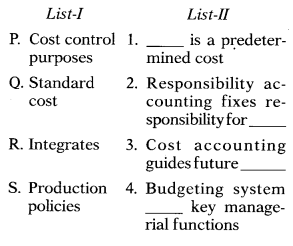Select the correct answer from the options given below ………..(B)

Question 227.
June 2016: A process in which management is looking outward to examine how others achieve their performance
levels and to understand the process they use is called —
(A) Balanced score card
(B) Target costing
(C) Bench marking process
(D) Performance analysis
(C) Bench marking process

Question 228.
June 2016: Match the following industry/product with appropriate cost unit:Select the correct answer using the codes given below —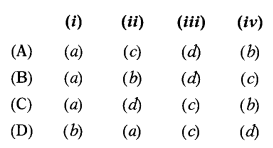(A)

Question 229.
June 2016: Which one of the following statements is false —
(A) Management accountant uses cost accounting tools and techniques for planning and decision making
(B) Management accounting is mostly historical in its approach and ill projects the past
(C) Cost accounting system can be installed without management accounting
(D) Management accounting focuses on wealth maximization
(B) Management accounting is mostly historical in its approach and ill projects the past

Question 230.
June 2016: Identify the cost which is not relevant or useful for decision making —
(A) Shut down cost
(B) Marginal cost
(C) Imputed cost and replacement cost
(D) Sunk cost
(D) Sunk cost

Question 231.
June 2016: Cost Accounting Standard is related to bringing uniformity and consistency in the principles and methods of determining the selling and distribution overheads with reasonable accuracy.
(A) 10
(B) 12
(C) 15
(D) 4
(C) 15

Question 232.
June 2016: Section of the Companies Act, 2013 gives the cost auditor same power as the financial auditor has under section of the Companies Act, 2013.
(A) 148, 143
(B) 143, 148
(C) 147, 148
(D) 143, 144
(A) 148, 143

Question 233.
June 2016: Those fixed costs which continue to be incurred even when there is no production are called —
(A) Period costs
(B) Discretionary costs
(C) Committed costs
(D) Output costs
(C) Committed costs

Question 234.
June 2016: A direct cost is a cost which can be classified on the basis of—
(A) Behaviour
(B) Traceability
(C) Controllability
(D) Relevance
(B) Traceability

Question 235.
June 2016: Management accounting does not include the function of —
(A) Planning and control
(B) Product costing
(C) Preparation of financial statements
(D) Decision-making
(D) Decision-making

Question 236.
Dec 2016: Which of the following is/ are tool(s) and technique(s) of management accounting?
(A) Ratio analysis
(B) Linear programming
(C) Trend analysis
(D) All of the above
(D) All of the above

Question 237.
Dec 2016: Match the following:Select the correct answer from the options given below —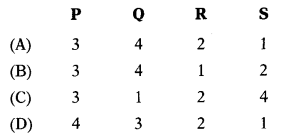(B)

Question 238.
Dec 2016: Statement -1
Sunk cost is one that has already been in-curred and cannot be avoided by decisions in the future.
Statement – II
For decision making, it is required that such cost should be incurred.
Select the correct answer from the options given below ………….
(A) Both statements are correct
(B) Both statements are incorrect
(C) Statement-I is incorrect, but Statement – I is correct
(D) Statement-I is correct, but Statement-II is incorrect
(D) Statement-I is correct, but Statement-II is incorrect

Question 239.
Dec 2016: The prime function of management accounting is to ………….
(B) Interpret financial data
(C) Assist the management in performing its functions effectively
(D) Assist tax authorities.
(C) Assist the management in performing its functions effectively

Question 240.
Dec 2016: Multiple costing is followed in………….
(A) Biscuit factory
(B) Steel industry
(C) Brick making
(D) Cycle manufacturing
(D) Cycle manufacturing

Question 241.
Dec 2016: The ascertainment of costs after they have been incurred is called ………….
(A) Marginal costing
(B) Historical costing
(C) Differential costing
(D) None of the above
(B) Historical costing

Question 242.
Dec 2016: A technique where standardized principles and methods of cost accounting are employed by a number of different companies is termed as —
(A) Uniform costing
(B) Absorption costing
(C) Standard costing
(D) ABC costing
(A) Uniform costing

Question 243.
Dec 2016: A cost centre is —
(A) A production or service location, function, activity or item of equipment whose costs may be attributed to cost units
(B) A centre for which an individual budget is drawn-up
(C) A centre where cost is classified on the basis of variability
(D) An amount of expenditure attributable to an activity-
(A) A production or service location, function, activity or item of equipment whose costs may be attributed to cost units

Question 244.
Dec 2016: In the management information system (MIS), top level management uses
(A) Operational information
(B) Tactical information
(C) Transactional information
(D) Strategic information
(D) Strategic information

Question 245.
Dec 2016: Which of the following is an irrelevant cost —
(A) Sunk cost
(B) Replacement cost
(C) Opportunity cost
(D) All of the above
(A) Sunk cost

Question 246.
Dec 2016: Match the following:
List -I List – II
(A) Advertising — (1) Operating costing
(B) Sugar company — (2) Job costing
(D) Transport — (4) Batch costing
Select the correct answer from the options given below —(A)

Question 247.
Dec 2014: In inflationary situation, which system of inventory valuation shows higher profits —
(A) LIFO
(B) FIFO
(C) HIFO
(D) Weighted average
(B) FIFO

Question 248.
June 2015: Which of the following method is based on the assumption that costliest materials are issued first and inventory is valued at the lowest possible price ……….
(A) FIFO method
(B) UFO method
(C) Highest-in-first-out method
(D) Weighted average method
(C) Highest-in-first-out method

Question 249.
June 2015: Following information is available regarding a product-X:
1st January, 2015:
Opening balance: 50 units @ 14 Receipts:
5th January, 2015: 100 units @ ₹ 5 12th January, 2015: 200 units @ ₹ 5.50 Issues: ‘
2nd January, 2015: 30 units 18th January, 2015:170 units
The value of closing stock according to FIFO method is —
(A) ₹ 660
(B) ₹ 770
(C) ₹ 825
(D) ₹ 860
(C) ₹ 825Question 250.
June 2015: In case of rising prices, FIFO method will provide—
(A) Lowest value of closing stock and profit
(B) Highest value of closing stock and profit
(C) Highest value of closing stock but lowest value of profit
(D) Lowest value of closing stock but highest value of profit
(B) Highest value of closing stock and profit

Question 251.
Dec 2015: FIFO method of valuing material issues is suitable in times of —
(A) Rising prices
(B) Falling prices
(C) Price fluctuation
(D) Boom period
(B) Falling prices

Question 252.
June 2016: XYZ Ltd. had 4,000 units of inventory in hand on 1st March, 2016, costing ₹ 4 per unit. Purchases and issues of material during the month were as follows:The cost of inventory as on 31st March, 2016 under FIFO and weighted average cost method will be
(A) ₹ 27,000 and ₹ 24,498
(B) ₹ 27,000 and ₹ 23,625
(C) ₹ 22,000 and ₹ 23,625
(D) ₹ 22,000 and ₹ 24,498
(A) ₹ 27,000 and ₹ 24,498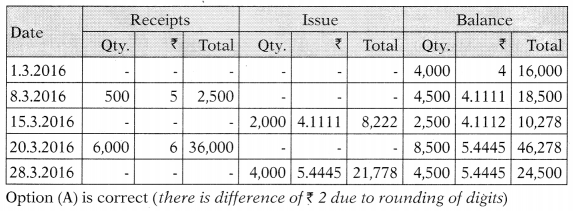Question 253.
Question June 2016: In a situation of rising prices, profit and tax liability would be lower under method than under method of material issue pricing.
(A) FIFO; LIFO
(B) LIFO; FIFO
(C) LIFO; Average
(D) FIFO; Average
(B) LIFO; FIFO

Question 254.
June 2016: The technique of economic order quantity is losing significance since the development of —
(A) Perpetual inventory
(B) Just-in-time
(C) First-in-first-out
(D) ABC analysis
(B) Just-in-time

Question 255.
June2016: Which one of the following is the correct sequence of the purchase procedure of inventory —
(A) Indenting for material, issuing tenders, receiving quotations, and placing order
(B) Issuing tenders and receiving quotations, indenting for material, and placing order
(C) Placing order, issuing tenders and receiving quotations, and indenting for material
(D) Indenting for material and placing order
(A) Indenting for material, issuing tenders, receiving quotations, and placing order

Question 256.
Dec 2016: Amaze Ltd. had an opening inventory of 5,000 units costing ₹ 5 per unit on 1st April, 2016. Following receipts and issues took place in April, 2016:
5th April, 2016: Purchased 800 units @ ₹ 8 per unit
12th April, 2016: Purchased 200 units @ ₹ 8 per unit
15th April, 2016: Issued 3,000 units 25th April, 2016: Purchased 1,000 units @ ₹ 9 per unit .
Cost of inventory as on 30th April, 2016 under weighted average basis will be —
(A) ₹ 25,500
(B) ₹ 27,000
(C) ₹ 20,000
(D) ₹ 23,500
(A) ₹ 25,500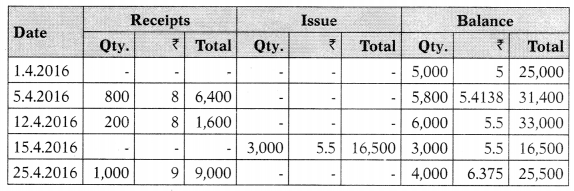Question 257.
Dec 2014: Which of the following is not a method used for time booking —
(A) Daily time sheets
(B) Weekly time sheets
(C) Job cards
(D) Pay roll
(D) Pay roll

Question 258.
June 2015: Which of the following is not an objective of time-booking —
(A) Apportionment of overheads against jobs
(B) Preparation of payrolls
(C) Ascertaining idle time for the purpose of control
(D) Calculation of labour cost of jobs done
(A) Apportionment of overheads against jobs

Question 259.
June 2016: Which of the following is/ are not a purpose of time keeping —
(i) Ascertaining labour cost of a job/ product/ activity
(ii) Evaluating labour performance by comparing actual and budgeted time
(iii) Providing internal check against dummy workers.
Select the correct answer from the options given below —
(A) (i) and (ii)
(B) (ii) and (iii)
(C) (i) and (iii)
(D) (ii) only
(A) (i) and (ii)

Question 260.
June 2016: Direct labour cost will include —
(A) All labour cost attributable to a production department
(B) Labour cost of production and production support services
(C) Cost of direct labour engaged in converting raw materials into manufactured articles
(D) Cost of labour recruited directly by the management and through contractors
(C) Cost of direct labour engaged in converting raw materials into manufactured articles

Question 261.
Dec 2016:
Statement -1
Low time wages do not necessarily mean low cost of production and high wages mean high cost of product.
Statement – II
Time and motion study, which is a function of engineering department, is useless for the determination of wages.
Select the correct answer from the options given below ………..
(A) Both statements are correct
(B) Both statements are incorrect
(C) Statement-I is incorrect, but Statement-I is correct
(D) Statement-I is correct, but Statement-II is incorrect
(D) Statement-I is correct, but Statement-II is incorrect

Question 262.
Dec 2014: Rent, rates and taxes paid for the building are apportioned on the basis of ………..
(A) Floor area
(B) Capital value
(C) No. of employees
(D) Direct labour hours
(A) Floor area

Question 263.
Dec 2014: If the actual expenses fall short of the amount absorbed, it is known as ………..
(A) Under absorption
(B) Over absorption
(C) Allocation
(D) Apportionment
(B) Over absorption

Question 264.
Dec 2014: The budgeted fixed over-heads amounted to ₹ 84,000. The budgeted and actual production amounted to 20,000 units and 24,000 units respectively. This means that there will be an —
(A) Under-absorption of ₹ 16,800
(B) Under-absorption of ₹ 14,000
(C) Over-absorption of ₹ 16,800
(D) Over-absorption of ₹ 14,000
(A) Under-absorption of ₹ 16,800
Actual overheads based on budgeted rate = $$\frac{84,000}{20,000} \times 24,000$$
20,000
Since budgeted overheads are less than actual overheads there is under absorption.
1,00,800- 84,000= 16,800.

Question 265.
June 2015: The following data is available for Akhil Ltd. for the year ended 31st March 2015:
Administrative overheads: ₹ 2,50,000 Production overheads: ₹ 2,74,200 Factory cost: ₹ 3,42,800 Work-in-progress: ₹ 74,000 Machine hour: 4,000 hours. The absorption rate for production over-heads is —
(A) ₹ 68.55
(B) ₹ 216.75
(C) ₹ 235.25
(D) ₹ 198.25
(A) ₹ 68.55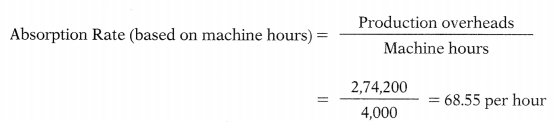Question 266.
June 2015: The budgeted fixed over-heads amounted to ₹ 75,000. The budgeted and Actual production amounted to 15,000 units and 20,000 units respectively. This means that there will be an —
(A) Under-absorption of ₹ 25,000
(B) Under-absorption of ₹ 18,750
(C) Over-absorption of ₹ 25,000
(D) Over-absorption of ₹ 18,750
(C) Over-absorption of ₹ 25,000
Absorption Rate = $$\frac{\text { Budgeted Overheads }}{\text { Budgeted Units }}=\frac{75,000}{15,000}=5 \text { per units }$$
Overhead absorbed = 20,000 units × 5 = 1,00,000. If it is assumed that actual overheads are same to that of budgeted figure then 1,00,000 – 75,000 = 25,000. Since absorbed overhead are more than actual overheads this is case of over absorption.

Question 267.
June 2015: The following data relates to two activity levels of production:
Level I Level II
No. of units 4,000 5,500
Variable cost per unit would be —
(A) ₹ 46.67
(B) ₹ 133.33
(C) ₹ 70
(D) ₹ 64
(A) ₹ 46.67
Variable Overhead Rate = $$\frac{\text { Change in Overheads }}{\text { Change in unit }}=\frac{70,000}{1,500}=46.67 \text { per unit }$$

Question 268.
June 2015: A product whose direct material costs and direct labour costs are ₹ 200 and ₹ 100 would consume 3 hours, 4 hours and 5 hours in department A, B & C respectively. Overheads absorption rate is – A: ₹ 4.5 per hour, B: ₹ 5 per hour and C: ₹ 10.5 per hour. The total cost of the product is —
(A) ₹ 486
(B) ₹ 386
(C) ₹ 214
(D) ₹ 500
(B) ₹ 386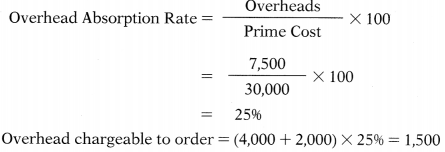Question 269.
Dec 2015: The following particulars relate to production department of a factory:
Material used : ₹ 20,000
Direct labour : ₹ 10,000
On an order carried out in the department, material consumed was ₹ 4,000 and direct wages paid amounted to ₹ 2,000. The amount of overheads chargeable to this order on the basis of prime cost would be –
(A) ₹ 1,500
(B) ₹ 1,510
(C) ₹ 1,700
(D) ₹ 1,710
(A) ₹ 1,500

Question 270.
Dec 2015: Allotment of the entire costs to a cost centre or unit is known as —
(A) Cost apportionment
(B) Cost allocation
(C) Cost absorption
(D) Machine hour rate
(B) Cost allocation

Question 271.
June 2016: Following information is given for an order:
Materials (direct) : ₹ 25,000
Wages (direct) : ₹ 20,000
Factory : 75% of wages (direct)
Sales : ₹ 85,800
Profit : 10% on cost of production
Office overheads are charged as a percentage of factory cost. The amount of office overheads and its percentage to factory cost will be —
(A) ₹ 78,000 and 30%
(B) ₹ 18,000 and 30%
(C) ₹ 25,800 and 43%
(D) ₹ 33,000 and 55%
(B) ₹ 18,000 and 30%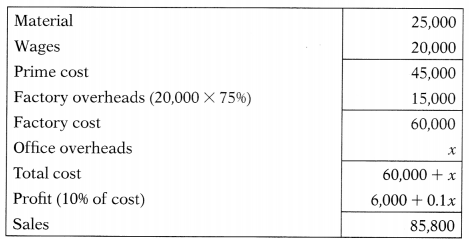60,000 + x + 6,000 + 0.1 = 85,800
66,000+ 1.1x = 85,800
1.1x = 19,800
x =18,000
%  of factory overheads to factory cost = $$=\frac{18,000}{60,000} \times 100=30 \%$$

Question 272.
June 2016: Which of the following statements are true —
(i) Conversion costs and overheads are interchangeable terms
(ii) Notional cost and imputed cost means the same thing
(iii) Cost accounting is not needed by a non-profit organization
(iv) Rent on owned building is included in cost accounts.
Select the correct answer from the options given below —
(A) (i) and (ii)
(B) (iii) and (iv)
(C) (ii) and (iv)
(D) All of the above
(A) (i) and (ii)

Question 273.
June 2016: Classify the following expenses as direct (D) and indirect (I) —
(i) Royalties charged as a rate per unit
(ii) Cost of making a design, pattern for a specific job
(iii) Salesman’s commission
(iv) Power, fuel, lighting of factory and office.
Select the correct answer using the codes given below —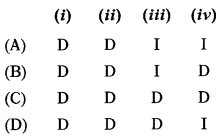(D)

Question 274.
Dec 2016: When the amount of overheads absorbed is less than the amount of overheads incurred, it is called —
(D) None of the above

Question 275.
Dec 2016:
Statement -1
Production departments and service departments are equally important for manufacturing industry.
Statement – II
To calculate cost of a product, service department cost should be redistributed among production department on a reasonable basis.
Select the correct answer from the options given below —
(A) Both statements are correct
(B) Both statements are incorrect
(C) Statement-I is incorrect, but Statement-II is correct
(D) Statement-I is correct, but Statement-II is incorrect
(A) Both statements are correct

Question 276.
Dec 2016: Following information has been collected from cost records of Bright Ltd.:
Direct material: ₹ 5,00,000 Direct labour: ₹ 3,00,000
Factory overheads: 20% of factory cost The amount of factory overheads will be:
(A) ₹ 1,60,000
(B) ₹ 2,00,000
(C) ₹ 1,80,000
(D) ₹ 1,96,000
(B) ₹ 2,00,000
20% of factory cost means 2596 of prime cost (5,00,000 + 3,00,000) × 2596 = 2,00,000.

Question 277.
Dec 2016: Labour hour rate Administrative overheads are absorbed on the basis of —
(A) Direct materials
(B) Direct wages
(C) Prime cost
(D) Works cost
(D) Works cost

Question 278.
Dec 2016: Following information relates to the production department of a factory:
Materials used : ₹ 80,000
Direct labour : ₹ 60,000
On an order carried out in the department, materials consumed amounted to ₹ 16,000. The overheads chargeable to this order on the basis of direct materials will be —
(A) ₹ 8,000
(B) ₹ 9,000
(C) ₹ 8,500
(D) ₹ 9,800
(A) ₹ 8,000
Overhead absorption rate = $$\frac{\text { Overheads }}{\text { Material }} \times 100=\frac{40,000}{80,000} \times 100=50 \%$$
Overheads to be absorbed for given order = 16,000 × 5096 = 8,000

Question 279.
Dec 2014: If selling price of a product is ₹ 85,800 and the profit margin on cost is 10%, the amount of profit will be —
(A) ₹ 7,800
(B) ₹ 8,580
(C) ₹ 7,200
(D) ₹ 9,533
(A) ₹ 7,800
Profit is 1096 on cost means 1 /11th of sale price.
Cost + Profit = Sales
100+10= 110
Profit = 85,800 $$\frac{10}{110}$$ = 7,800

Question 280.
Dec 2014: Which of the following is to be included while preparing a cost sheet —
(A) Interest paid
(B) Goodwill written-off
(C) Income-tax paid
(D) Salesman commission
(D) Salesman commission

Question 281.
Dec 2014: Cost of production plus opening stock of finished goods minus closing stock of finished goods is equal to—
(A) Cost of goods sold
(B) Cost of sales
(C) Sales
(D) Prime cost
(A) Cost of goods sold

Question 282.
Dec 2014: Which of the following is not a type of job costing —
(A) Terminal costing
(B) Contract costing
(C) Batch costing
(D) Operation costing
(D) Operation costing

Question 283.
June 2015:
Net works cost: ₹ 3,00,000 Administrative overheads: ₹ 1,00,000 Opening stock of finished goods: Nil Closing stock of finished goods: ₹ 20,000 Selling overheads: ₹ 10,000. From the above information, the cost of sales will be —
(A) 4,30,000
(B) 3,90,000
(C) 3,70,000
(D) 4,10,000
(B) 3,90,000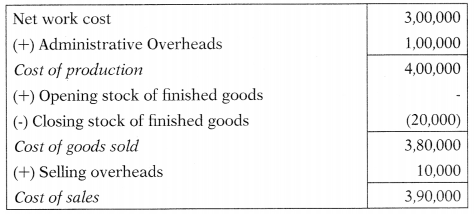Question 284.
June 2015: Following information is available regarding an organization:
Direct material purchased: ₹ 1,50,000 Direct material consumed: ₹ 80,000 Direct labour: ₹ 50,000 Direct expenses: ₹ 30,000 Manufacturing overheads: ₹ 20,000 The prime cost for the organization is—
(A) 1,60,000
(B) 2,90,000
(C) 2,30,000
(D) 1,80,000
(A) 1,60,000

Question 285.
June 2015: If the sales of a product is ₹ 94,080 and the profit margin on cost 12%, the amount of profit will be ………………
(A) ₹ 7,800
(B) ₹ 11,290
(C) ₹ 8,580
(D) ₹ 10,080
(D) ₹ 10,080
Cost + Profit = Sales
100 +12 = 112
Profit = 94,080 × $$\frac{12}{112}$$ = 10,080

Question 286.
Dec 2015: Item(s) excluded from cost sheet are —
(A) Direct material
(C) Provision for taxation
(D) All of the above
(C) Provision for taxation

Question 287.
Dec 2015: The following information is extracted from the job ledger in respect of Job No. 404:
Material: ₹ 3,400
Wages: 80 hours @ ₹ 2.50 per hour
Variable overheads incurred for all jobs: ₹ 5,000 for 4,000 labour hours
If the job is billed for ₹ 4,200 the profit will be —
(A) ₹ 600
(B) ₹ 500
(C) ₹ 700
(D) ₹ 650
(B) ₹ 500Question 288.
June 2016: From the following particulars relating to Job No. 555, ascertain the total cost:
Direct materials 16,000
Direct labour 8,000
Direct expenses 1,600
Works overheads are recovered on the basis of 50% on prime cost and administrative overheads at 10% of works cost. Choose the correct option —
(A) 45,000
(B) 45,240
(C) 42,240
(D) 43,000
(C) 42,240Question 289.
June 2016: Direct material cost ₹ 45,000; Direct labour cost is 40% of direct material cost; Royalties on production ₹ 4,000; Other direct expenses are 20% of prime cost. Prime cost will be —
(A) ₹ 78,750
(B) ₹ 83,750
(C) ₹ 80,400
(D) None of the above-
(B) ₹ 83,75045,000 + 18,000 + 4,000 + 0.2
67,000+ 0.2x=x
67,000 = 0.8x
x = 83,750

Question 290.
June 2016:
Cost of production for 10,000 units: ₹ 1,60,000
Opening stock of finished goods (1,000 units) : ₹ 18,000
Closing stock of finished goods (FIFO) : 2,000 units
Selling & distribution overheads : ₹ 2 per unit sold
Profit mark-up on selling : 20% price
The amount of profit will be —
(A) ₹ 39,800
(B) ₹ 40,500
(C) ₹ 41,000
(D) ₹ 40,800
(C) ₹ 41,000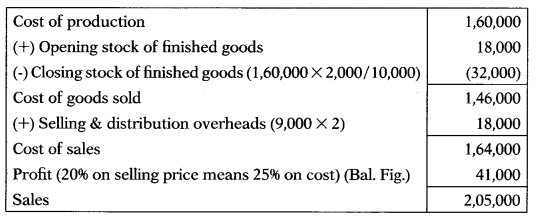Question 291.
Dec 2016: Following information is given:
Direct material purchased : 6,00,000
Direct material consumed : 7,00,000
Direct labour : 3,00,000
Direct expenses : 2,50,000
Prime cost will be —
(A) ₹ 14,50,000
(B) ₹ 11,50,000
(C) ₹ 12,50,000
(D) ₹ 15,50,000